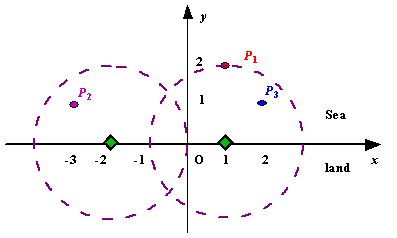poj1328,

## Problem:

 Time Limit: 1000MS Memory Limit: 10000K Total Submissions: 24047 Accepted: 5156
Description
Assume the coasting is an infinite straight line. Land is in one side of coasting, sea in the other. Each small island is a point locating in the sea side. And any radar installation, locating on the coasting, can only cover d distance, so an island in the sea can be covered by a radius installation, if the distance between them is at most d.

We use Cartesian coordinate system, defining the coasting is the x-axis. The sea side is above x-axis, and the land side below. Given the position of each island in the sea, and given the distance of the coverage of the radar installation, your task is to write a program to find the minimal number of radar installations to cover all the islands. Note that the position of an island is represented by its x-y coordinates.Figure A Sample Input of Radar Installations

Input
The input consists of several test cases. The first line of each case contains two integers n (1<=n<=1000) and d, where n is the number of islands in the sea and d is the distance of coverage of the radar installation. This is followed by n lines each containing two integers representing the coordinate of the position of each island. Then a blank line follows to separate the cases.

The input is terminated by a line containing pair of zeros
Output
For each test case output one line consisting of the test case number followed by the minimal number of radar installations needed. "-1" installation means no solution for that case.
Sample Input
```3 2
1 2
-3 1
2 1

1 2
0 2

0 0```
Sample Output
```Case 1: 2
Case 2: 1```
Source
Beijing 2002

## Solution:

Basically,
1. it converts the position of radar to the segment in the line.
2. find out the minimum number of radars by using Greedy algorithm.
PS: for greedy algorithm.
1. keep the right most position of the current radar.
2. if the new left position is greater than current kept position, then number of radar need to increase.
3. if the new right position if less than current position we kept, then move back a little bit to cover this new island.
Faint: Don't forget to check N, the program will stop if it's 0;

## Source Code:

//Tue Apr 13 14:01:31 CDT 2010
#include <vector>
#include <list>
#include <map>
#include <set>
#include <deque>
#include <queue>
#include <stack>
#include <bitset>
#include <algorithm>
#include <functional>
#include <numeric>
#include <utility>
#include <sstream>
#include <iostream>
#include <iomanip>
#include <cstdio>
#include <cmath>
#include <cstdlib>
#include <cctype>
#include <string>
#include <cstring>
#include <cstdio>
#include <cmath>
#include <cstdlib>
#include <ctime>

using namespace std;

#define eps 10e-7
{
public:
double left;
double right;
};

{
return A.left < B.left;
}

int main(int argc, const char* argv[])
{
freopen("input.in", "r", stdin);
freopen("output.out", "w", stdout);
int N, d;
for (int ncase = 1; (cin >> N >> d) && N; ncase++)
{
bool flag = true;
v.clear();
for (int i = 0; i < N; i++)
{
int x, y;
cin >> x >> y;
if (y > d)
flag = false;
else
{
double tmp = sqrt(1.0 * d * d - 1.0 * y * y);
r.left = 1.0 * x - tmp;
r.right = 1.0 * x + tmp;
v.push_back(r);
}

}
if (flag == false)
{
cout << "Case " << ncase << ": " << -1 << endl;
continue;
}
sort(v.begin(), v.end(), cmp);
int number = 1;
double preidx = v.right;
for (int i = 1; i < N; i++)
{
if (v[i].left > preidx + eps)
{
number++;
preidx = v[i].right;
}
else
{
if (preidx > v[i].right + eps)
preidx = v[i].right;
}
}
cout << "Case " << ncase << ": " << number << endl;
}
fclose(stdin);
fclose(stdout);
return 0;
}